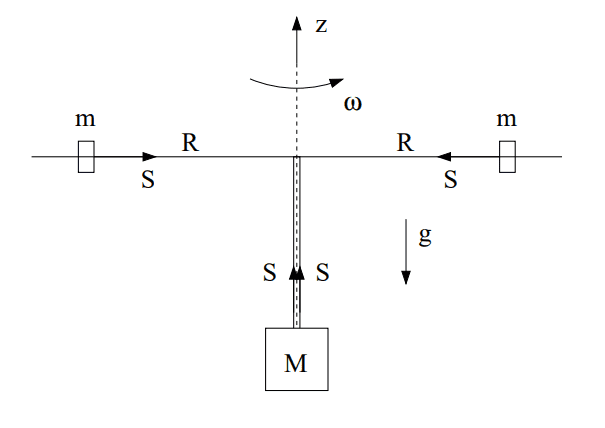# Two rotating masses balanced by a third mass (rotational dynamics)

Eirik
Homework Statement:
Hi! This isn't really homework, but I'm practicing for my exam in mechanical physics and I'm really struggling with this one question!

Two masses, m, are rotating around the z axis as shown in the figure on a frictionless surface, but are being pulled down by a third mass, M. Find the differential equation for the movement of the system. The masses should be considered point masses and the system initially has ##R=R_0## and ##\omega=\omega_0## It should be on the following form, where ##\alpha## and ##\beta## are constants you need to find:
Relevant Equations:
##\ddot R + \alpha g - \frac{\beta}{R^3}=0##, differential equation I need to find

##a_r = \ddot R -\omega^2R##, acceleration of mass m in the circle, given in task
Here's a diagram of what the system looks like:So far I have figured out what the initial angular velocity is, if the system is balanced (no movement):

## \sum F_m = m*\frac{v^2}{R_0}-\frac{Mg}{2}=0 ##
##m \frac{v^2}{R_0}-\frac{Mg}{2}=0 ## divide both sides by m
##\omega_0 = \sqrt{\frac{Mg}{2mR_0}}##

One of the hints given for the task, was that we should consider the conservation of angular momentum:
Moment of inerty, start: ##I_0=2mR_0^2##
Angular momentum, start: ##L_0=I_0\omega_0=2mR_0^2 \sqrt{\frac{Mg}{2mR_0}} ##

I am struggling to find the equation for the angular momentum after that, however. I know that the moment of inertia should still be ##I=2mR^2##, and that ##L=I\omega##. How do I find \omega?

I also have this:
## \sum F_M = Mg-2S=M*\ddot R##
##S=m* (\ddot R -\omega^2R)## Really not sure if this one is correct

Any help would be very greatly appreciated!

Homework Helper
Gold Member
Homework Statement::
So far I have figured out what the initial angular velocity is, if the system is balanced (no movement):
If the system were to start out in the balanced state, then it would remain in this state. I don't think they want you to assume the system is in the balanced state initially. I think they want you to express the differential equation for ##\ddot R## in terms of ##R_0## and ##\omega_0##.

One of the hints given for the task, was that we should consider the conservation of angular momentum:
Moment of inerty, start: ##I_0=2mR_0^2##
Angular momentum, start: ##L_0=I_0\omega_0=2mR_0^2 \sqrt{\frac{Mg}{2mR_0}} ##
Since the system does not necessarily start in the balanced state, you cannot assume that ##\omega_0=\sqrt{\frac{Mg}{2mR_0}} ##. Just express ##L_0## in terms of ##m##, ##R_0##, and ##\omega_0##.

I am struggling to find the equation for the angular momentum after that, however. I know that the moment of inertia should still be ##I=2mR^2##, and that ##L=I\omega##.
Yes.

How do I find ##\omega##?
You can use the expression for the angular momentum to find ##\omega## as a function of ##R##.

I also have this:
## \sum F_M = Mg-2S=M*\ddot R##
Be careful with signs. The left side expresses the net downward force on ##M##. So, the acceleration on the right should be the downward acceleration of ##M##. Does the downward acceleration of ##M## equal ##\ddot R## or ##-\ddot R##?

##S=m* (\ddot R -\omega^2R)##
You have a sign error in this equation. Does the force ##S## on ##m## act in the radially outward direction or the radially inward direction?

Eirik
Thank you so much @TSny ! That was really, really helpful!

Then we have
##L_i=L##
##2mR_0^2\omega_0=2mR^2\omega##
Solving for ##\omega## gives us ##\omega=\frac{R_0^2\omega_0}{R^2}##

And if we choose the positive direction for the accelaration along -z, it should be ##S=m*(\omega^2R-\ddot R)## instead, as the direction for m's acceleration will be radially inward. If I now substitute ##\omega## in this expression, I get:

##S=m*((\frac{R_0^2\omega_0}{R^2})^2R-\ddot R) = m(\frac{R_0^4\omega_0^2}{R^3}-\ddot R)##

Substitution in ##Mg-2S=M\ddot R## gives us:

##Mg-2(m(\frac{R_0^4\omega_0^2}{R^3}-\ddot R))=M\ddot R##

And after doing some algebra I get:

##\ddot R - \frac{M}{M-2m}g + \frac{\frac{2mR_0^4\omega_0^2}{M-2m}}{R^3}=0##

Meaning that ##\alpha = \frac{M}{M-2m}## and ##\beta = \frac{2mR_0^4\omega_0^2}{M-2m}##

Yay!Does that look right?

Homework Helper
Gold Member
Everything looks good up to here:
Substitution in ##Mg - 2S = M \ddot R##
This equation has a sign error. Review the comments near the end of post #2. This will alter your final result. Otherwise, I think you have it.

Eirik
@TSny Yes, of course! 🤦‍♂️ I thought ##\ddot R## would be M's downward acceleration, but R will get smaller over time, so it has to be ##Mg-2S=-M\ddot R##

That finally gives me

##\ddot R + \frac{M}{M+2m}g - \frac{\frac{2mR_0^4\omega_0^2}{M+2m}}{R^3}=0##

with ##\alpha = \frac{M}{M+2m}## and ##\beta = \frac{2mR_0^4\omega_0^2}{M+2m}##

That also checks out with the signs the task said I was supposed to end up with lol

Thank you again so, so much for all the help!

•TSny